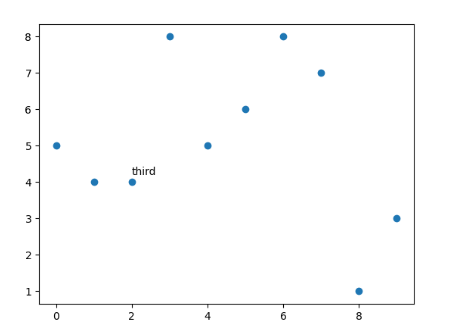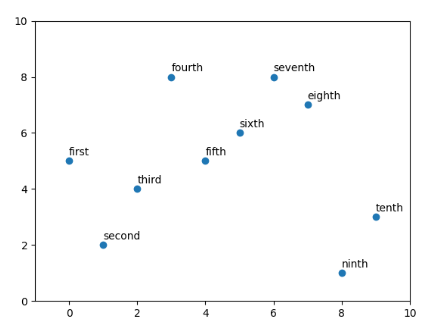Related Articles
How to Annotate Matplotlib Scatter Plots?
• Last Updated : 24 Feb, 2021

A scatter plot uses dots to represent values for two different numeric variables. In Python, we have a library matplotlib in which there is a function called scatter that helps us to create Scatter Plots. Here, we will use matplotlib.pyplot.scatter() method to plot.

Syntax : matplotlib.pyplot.scatter(x,y)

Parameters:

• x and y are float values and are the necessary parameters to create a scatter plot
• marker : MarkerStyle, default: rcParams[“scatter.marker”] (default: ‘o’)
• cmap : cmapstr or Colormap, default: rcParams[“image.cmap”] (default: ‘viridis’)
• linewidths : float or array-like, default: rcParams[“lines.linewidth”] (default: 1.5)
• alpha : float, default: None → represents the transparency

Annotation of matplotlib means that we want to place a piece of text next to the scatter. There can be two cases depending on the number of the points we have to annotate :

1. Single point annotation
2. All points annotation

### Single Point annotation

In single-point annotation we can use matplotlib.pyplot.text and mention the x coordinate of the scatter point and y coordinate + some factor so that text can be distinctly visible from the plot, and then we have to mention the text.

Syntax: matplotlib.pyplot.text( x, y, s)

Parameters:

• x, y : scalars — The position to place the text. By default, this is in data coordinates. The coordinate system can be changed using the transform parameter.
• s : str — The text.
• fontsize — It is an optional parameter used to set the size of the font to be displayed.

Approach:

1. Import libraries.
2. Create data.
3. Make scatter plot.
4. Apply plt.text() method.

Implementation:

## Python3

 `# Importing libraries``import` `matplotlib.pyplot as plt`` ` `# Preparing dataset``x ``=` `[x ``for` `x ``in` `range``(``10``)]``y ``=` `[``5``, ``4``, ``4``, ``8``, ``5``, ``6``, ``8``, ``7``, ``1``, ``3``]`` ` `# plotting scatter plot``plt.scatter(x, y)`` ` `# annotation of the third point``plt.text(``2``,``4.2``,``"third"``)``plt.show()`

Output:### All points annotation

If we want to annotate all points in the scatter plot then matplotlib.pyplot has an inbuilt function annotate which takes the text, x, and y coordinates of the point.

Syntax: matplotlib.pyplot.annotate( text, xy )

Parameters:

• text : str — The text of the annotation. s is a deprecated synonym for this parameter.
• xy : (float, float) — The point (x, y) to annotate. The coordinate system is determined by xy coordinates.

Approach:

1. Import libraries.
2. Create data.
3. Store all the annotations in a list in order with the sequence of the points to be displayed.
4. Draw the scatter plot.
5. Using a for loop annotate each point.

Implementation:

## Python3

 `# Importing libraries``import` `matplotlib.pyplot as plt`` ` `# Preparing dataset``x ``=` `[x ``for` `x ``in` `range``(``10``)]``y ``=` `[``5``, ``2``, ``4``, ``8``, ``5``, ``6``, ``8``, ``7``, ``1``, ``3``]``text ``=` `[``"first"``, ``"second"``, ``"third"``, ``"fourth"``, ``"fifth"``,``        ``"sixth"``, ``"seventh"``, ``"eighth"``, ``"ninth"``, ``"tenth"``]`` ` `# plotting scatter plot``plt.scatter(x, y)`` ` `# Loop for annotation of all points``for` `i ``in` `range``(``len``(x)):``    ``plt.annotate(text[i], (x[i], y[i] ``+` `0.2``))`` ` `# adjusting the scale of the axes``plt.xlim((``-``1``, ``10``))``plt.ylim((``0``, ``10``))``plt.show()`

Output:Attention geek! Strengthen your foundations with the Python Programming Foundation Course and learn the basics.

To begin with, your interview preparations Enhance your Data Structures concepts with the Python DS Course.

My Personal Notes arrow_drop_up